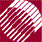ECE532 Biomedical Optics © 1998 Steven L. Jacques, Scott A. Prahl Oregon Graduate Institute

# Diffusion theory

## Fick's 1st law of diffusion

Diffusion occurs in response to a concentration gradient expressed as the change in concentration due to a change in position,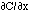. The local rule for movement or flux J is given by Fick's 1st law of diffusion: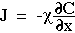in which the flux J [cm-2 s-1] is proportional to the diffusivity[cm2/s] and the negative gradient of concentration,[cm-3 cm-1] or [cm-4]. The negative sign indicates that J is positive when movement is down the gradient, i.e., the negative sign cancels the negative gradient along the direction of positive flux.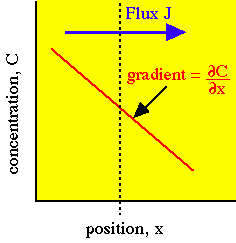The flux J is driven by the negative gradientin the direction of increasing x.

### Example: arbitrary units

Consider a local concentration of 106 units per cm3 which drops by 10% over a distance of 1 cm. Then the gradient is -105 [units cm-4]. Assume the diffusivity is 103 [cm2/s]. Then the flux J equals:

J = -(103 [cm2/s])(-105 [units cm-4]) = 108 units cm-2 s-1

For light, the diffusivity is proportional to the diffusion length D [cm] and the speed of light c:

##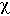= cD

where D = 1/(3 µs(1-g)). The units of velocity [cm/s] times the units of length [cm] yield the units of diffusivity [cm2/s]. The following example describes the local diffusion of red light in milk.

### Example: optical energy

Consider a local fluence rate F of 1 W/cm2 in milk (n = 1.33, µs(1-g) = 10 cm-1).

• The local concentration of optical energy is C = F/c = (1 W/cm2)/(2.2x1010 cm/s) = 4.4x10-11 J cm-3.
• Assume the concentration drops by 10% over a distance of 3 mm. Then the gradientis -(0.10)(4.4x10-11 cm-3)/(0.3 cm) = -1.5x10-11 J cm-4.
• The diffusivityequals cD where D = 1/(3(10 cm-1)) = 0.033 cm. Therefore= (2.2x1010 cm/s)(0.033 cm) = 7.5x1010 cm2/s.
• Then the flux J along the direction x equals -= -(7.5x1010 cm2/s)(-1.5x10-11 J cm-4) = 0.11 J cm-2 s-1 = 0.11 W cm-2.

For optical diffusion, Fick's 1st law is expressed as the energy flux J [W cm-2] proportional to the diffusion constant D [cm] and the negative fluence gradient dF/dx: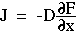which was obtained by substituting cD forand substituting F/c for C. The factors c and 1/c cancel to yield the above equation.

to next page | Chapter 5 | Course | Home